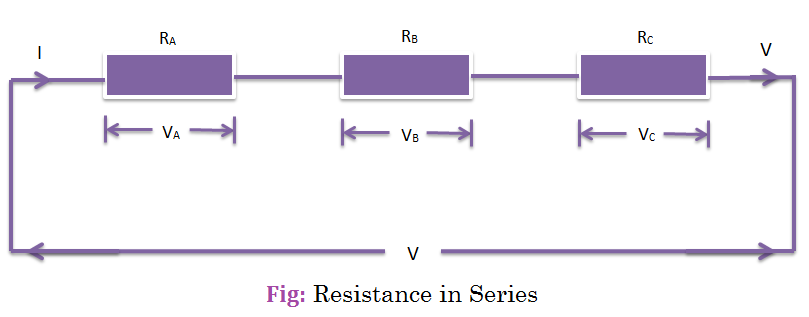# Resistance in Series and Parallel

## Resistance in Series:

When the resistance of the conductor are joined end to end, they are called Series grouping.Let RA, RB and RC are connected in Series and VA,VB and VC be the voltage-drops in these corresponding resistance and circuit-current is ‘I’ which is constant in series circuit:

Now, V= VA+VA+VA=IRA+IRB+IRC
V=I(RA+RB+RC)
or, V/I= RA+RB+RC = R = Total or equivalent resistance of the circuit.

## Resistance in Parallel:

if the similar ends of each resistance are joined to a common point, and at the other ends, other resistors are joined to another common point then it is said to be a Parallel grouping of resistance.Let RA and RB be the two resistors which are joined in parallel and corresponding current be IA, IB and ‘V’ be the voltage applied across each resistor and voltage is constant in parallerl circuit.

Now, circuit ‘I’ = IA + IB
Again, I = (V/RA) + (V/RB) (applying Ohm’s Law)
=V(1/RA) + (1/RB)
or, I/V= (1/RA) + (1/RB)=1/R
where R= Total or equivalent resitance of the circuit.